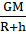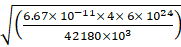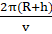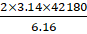SSC BOARD PAPERS IMPORTANT TOPICS COVERED FOR BOARD EXAM 2024

### Solve the following problems. How much time a satellite in an orbit at height 35780 km above earth’s surface would take, if the mass of the earth would have been four times its original mass?

Question 10.

Solve the following problems.

How much time a satellite in an orbit at height 35780 km above earth’s surface would take, if the mass of the earth would have been four times its original mass?

Given G = gravitational constant = 6.67 x 10-11 N m2/kg2

M mass of earth = 4 x 6 x 1024 kg

R = 6400 km

h = height of satellite above earth’s surface = 35780 km

R+h = 6400 + 35780 = 42180 km = 42180 x 103 m

v = ?

we know

v = √=v = 6.16 km/s

We know v = distance/time

= circumference/time

= 2πr/T

T =T =T = 43001.68 sec

T = 11.94 hr or approximately 12 hours.